# Convolutional Neural Network (CNN): Graphical Visualization with Code Explanation

• Tanesh Balodi
• Sep 06, 2019
• Deep LearningConvolutional neural networks are neural networks that are mostly used in image classification, object detection, face recognition, self-driving cars, robotics, neural style transfer, video recognition, recommendation systems, etc.

CNN classification takes any input image and finds a pattern in the image, processes it and classifies it in various categories which are like Car, Animal, Bottle, etc. CNN is also used in unsupervised learning for clustering images by similarity. It is a very interesting and complex algorithm, which is driving the future of technology.

## What is Convolutional Neural Network (CNN)?

Convolution neural networks” indicates that these are simply neural networks with some mathematical operation (generally matrix multiplication) in between their layers called convolution.

It was proposed by Yann LeCun in 1998. It's one of the most popular uses in Image Classification. Convolution neural network can broadly be classified into these steps :

1. Input layer

2. Convolutional layer

3. Output layer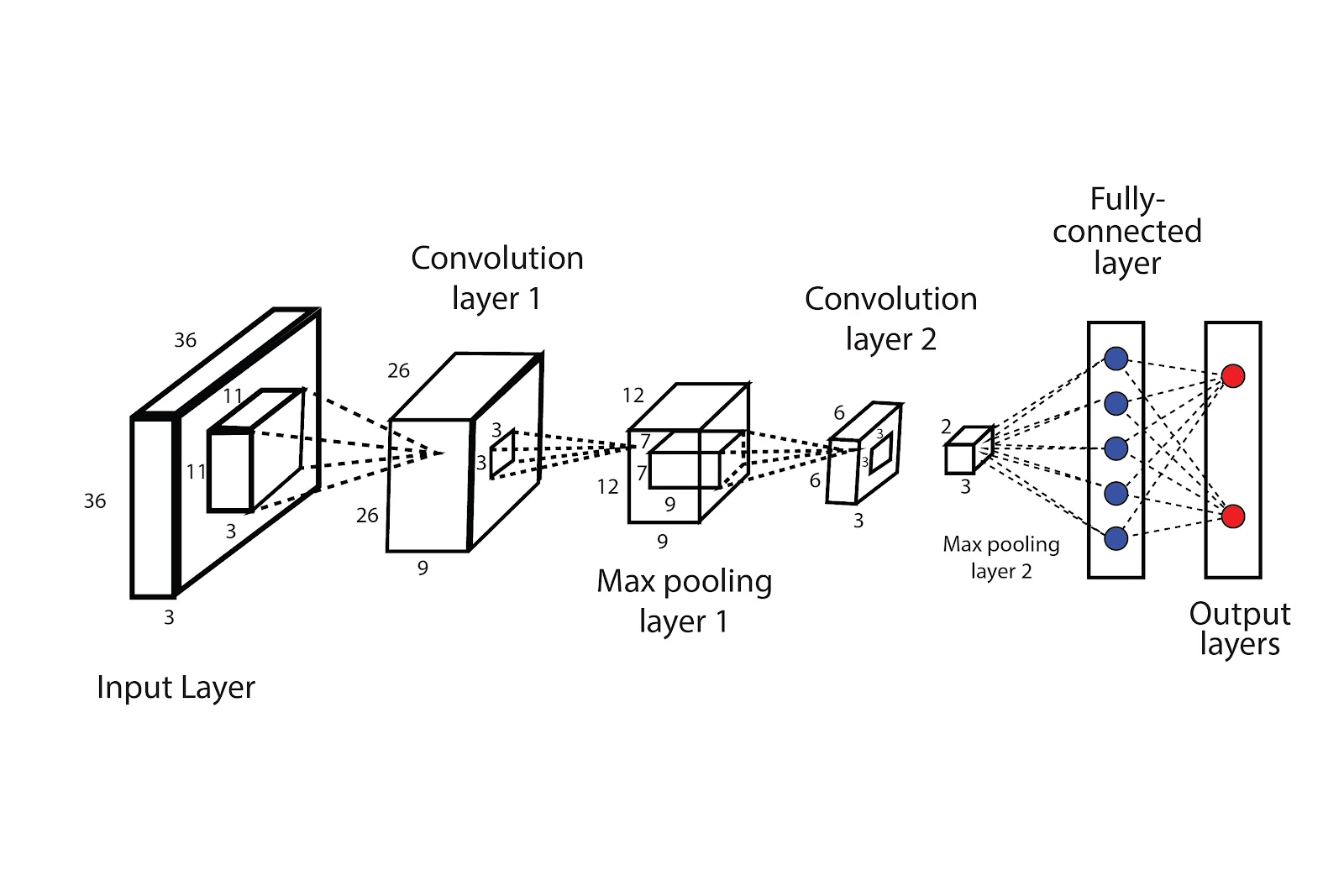The architecture of Convolutional Neural Networks(CNN)

Input layers are connected with convolutional layers which perform many tasks such as padding, striding, functioning of kernels for so many performances of this layer, this layer is considered as a building block of convolutional neural networks.

(Speaking of convolutional neural networks, you can also check out our blog on Introduction to Common Architectures in Convolution Neural Networks)

We will be discussing it’s functioning and how the fully connected networks work.

## Introduction of Convolutional Layer and Max-pooling Layer

Convolutional layer’s main objective is to extract features from images, and learn learns all the features of the image which would help in object detection techniques. As we know, the input layer will contain some pixel values with some weight and height, our kernels or filters will convolve around input layer and give results which will retrieve all the feature with fewer dimensions. Let’s see how kernels work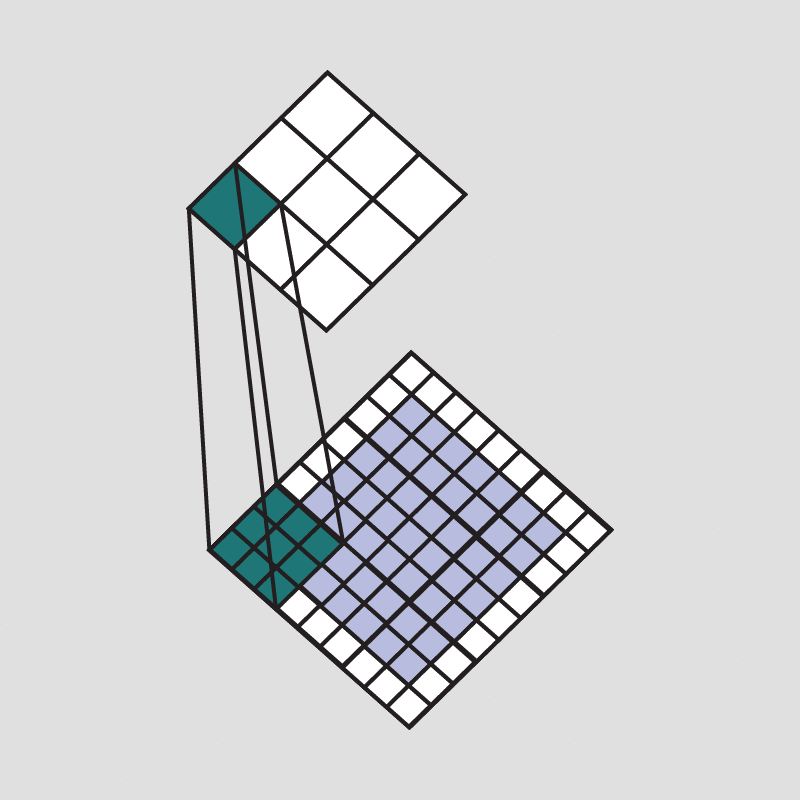Formation and arrangement of Convolutional Kernels

With the help of this very informative visualization about kernels, we can see how the kernels work and the how padding is done.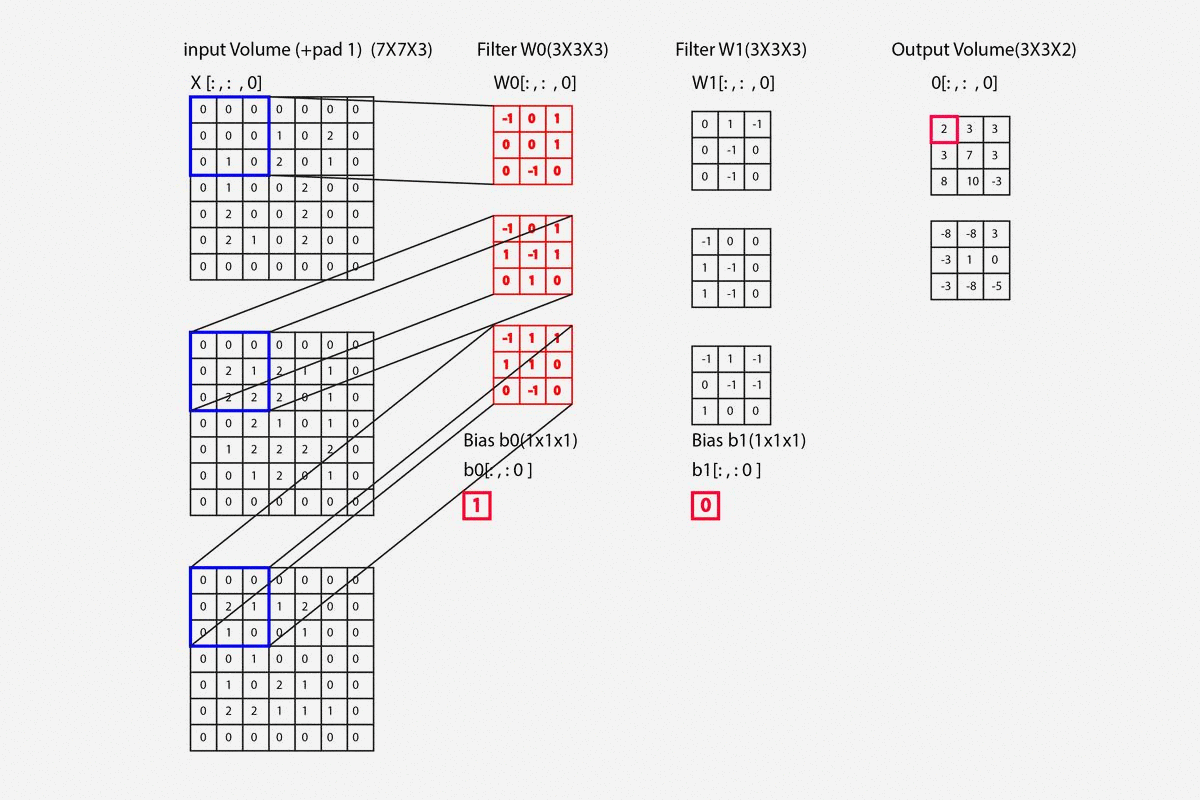Matrix visualization in CNN

We can see padding in our input volume, we need to do padding in order to make our kernels fit the input matrices. Sometimes we do zero paddings, i.e. adding one row or column to each side of zero matrices or we can cut out the part, which is not fitting in the input image, also known as valid padding.

Let’s see how we reduce parameters with negligible loss, we use techniques like Max-pooling and average pooling.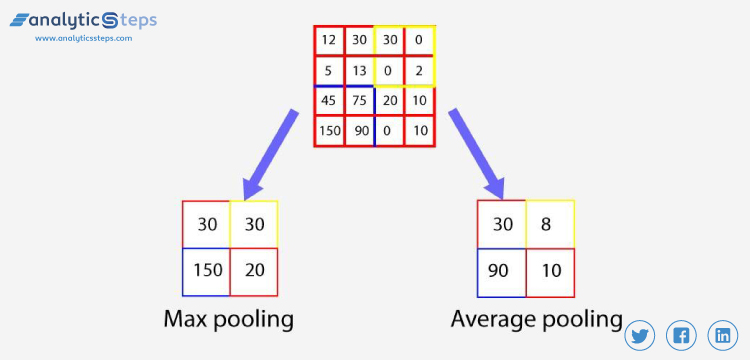Matrix formation using Max-pooling and average pooling

Max pooling or average pooling reduces the parameters to increase the computation of our convolutional architecture. Here, 2*2 filters and 2 strides are taken (which we usually use). By name, we can easily assume that max-pooling extracts the maximum value from the filter and average pooling takes out the average from the filter. We perform pooling to reduce dimensionality. We have to add padding only if necessary. The more convolutional layer can be added to our model until conditions are satisfied.

## Applying the Activation Function

An activation function is added to our network anywhere in between two convolutional layers or at the end of network, so you must be wondering what exactly an activation function does, let me clear it in simple words for you, it helps in making decision about which information should fire forward and which not by making decisions at the end of any network.

In broadly, there are both linear as well as non-linear activation functions, both performing linear and non-linear transformations but non-linear activation functions is a lot helpful and therefore widely used in neural networks as well as deep learning networks.

Four most famous activation functions to add non-linearity to the network are described below.### Sigmoid Activation Function

The equation for the sigmoid function is

f(x) = 1/(1+e-X )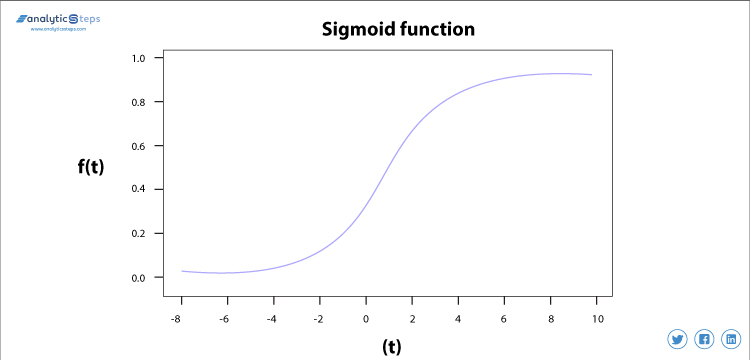Sigmoid Activation function

The sigmoid activation function is used mostly as it does its task with great efficiency, it basically is a probabilistic approach towards decision making and ranges in between 0 to 1, so when we have to make a decision or to predict an output we use this activation function because of the range is the minimum, therefore, prediction would be more accurate.

### Hyperbolic Tangent Activation Function(Tanh)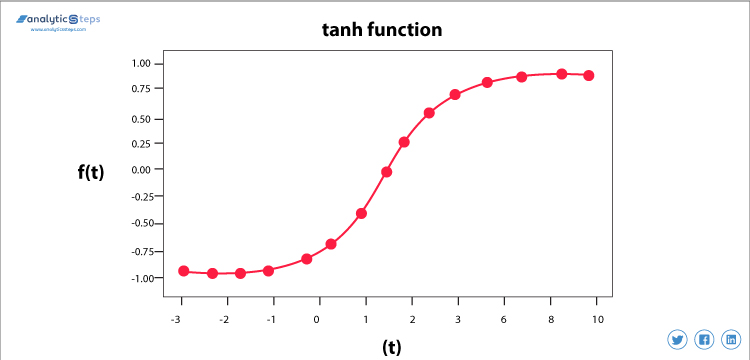Tanh Activation function

This activation function is slightly better than the sigmoid function, like the sigmoid function it is also used to predict or to differentiate between two classes but it maps the negative input into negative quantity only and ranges in between -1 to  1.

### ReLU( Rectified Linear unit) Activation function

Rectified linear unit or ReLU is most widely used activation function right now which ranges from 0 to infinity, All the negative values are converted into zero, and this conversion rate is so fast that neither it can map nor fit into data properly which creates a problem, but where there is a problem there is a solution,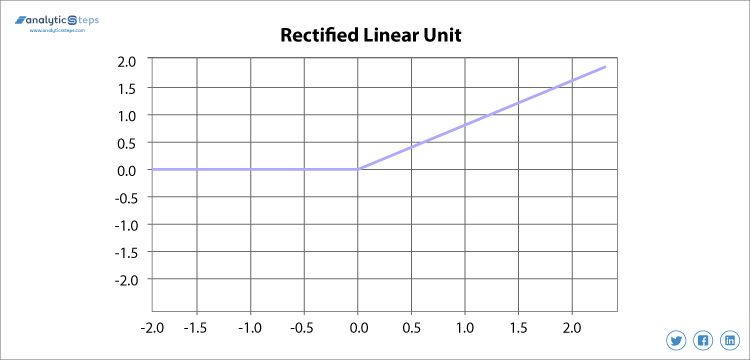Rectified Linear Unit activation function

we use Leaky ReLU function instead of ReLU to avoid this unfitting, in Leaky ReLU range is expanded which enhances the performance.

### Softmax Activation Function

Softmax is used mainly at the last layer i.e output layer for decision making the same as sigmoid activation works, the softmax basically gives value to the input variable according to their weight and sum of these weights is eventually one.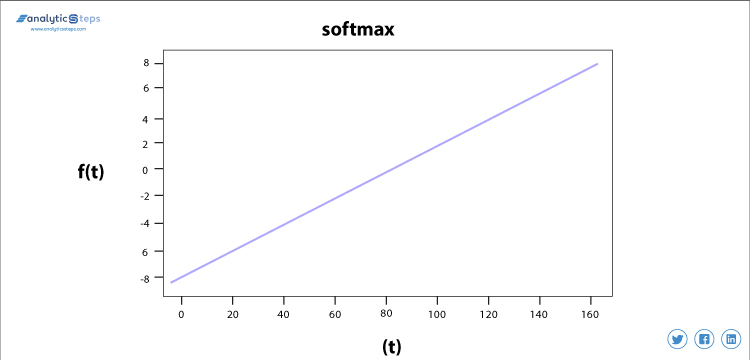Softmax activation function

For Binary classification, both sigmoid, as well as softmax, are equally approachable but in case of multi-class classification problem we generally use softmax and cross-entropy along with it.

Our next step would be a Fully Connected Network (FCN)View to Fully Connected Network (FCN)

Our last layer which is connected is fully connected network and we will be sending our flatten data to a fully connected network, we basically transform our data to make classes that we require to get from our network as an output.

## Let’s see the code for the Convolutional Neural Network

Step 1: Importing all necessary libraries(mainly from Keras)Importing sequential model, activation, dense, flatten, max-pooling libraries.

Step 2: Importing dataset. If you want to use the same dataset you can download.Step 3 :Visualizing our dataset and splitting into training and testing. Here, np.utils converts a class integer to the binary class matrix for use with categorical cross-entropy.

Step 4 :Reshaping our x_train and x_test for use in conv2D. And we can observe the change in the shape of our data.

Step 5 :This is the main structural part of CNN, where CNN is implemented, we have taken two convolutional layers and we can see we have added different activation functions like relu, sigmoid, and softmax. Our structure goes in accordance with what we have already discussed above.

Step 6 :To compute loss, we use categorical cross-entropy, for more functionality of Keras, you can visit the documentation of Keras from keras.org.

Step 7 :Fitted our training data to our model and took the batch size as 128, which will take 128 values at once till total parameters are satisfied. Here epochs means the number of times it will be processed.

Step 8 :The plot for loss between the training set and testing set.The plot for accuracy on the training set and test set has been visualised with the help of matplotlib.

## Conclusion

CNN is the best artificial neural network technique, it is used for modeling images but it is not limited to just modeling of the image but out of many of its applications, there is some real-time object detection problem which can be solved with the help of this architecture. There are many improvised versions based on CNN architecture like AlexNet, VGG, YOLO and many more. For more blogs in Analytics and new technologies do read Analytics Steps.

0%### Tanesh BalodiA splendid and inventive Machine Learning Intern in Analytics Steps. He loves deploying data in ML and finds out different insights to play with industry trends.

### Trending blogs

• What is the OpenAI GPT-3?

• Introduction to Time Series Analysis: Time-Series Forecasting Machine learning Methods & Models

• How is Artificial Intelligence (AI) Making TikTok Tick?

• 6 Major Branches of Artificial Intelligence (AI)

• 7 Types of Activation Functions in Neural Network

• 7 types of regression techniques you should know in Machine Learning

• Top 10 Big Data Technologies in 2020